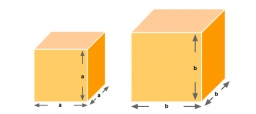# Area to volume

If the surface area of a cube is 486, find its volume.

V =  729

### Step-by-step explanation:Did you find an error or inaccuracy? Feel free to write us. Thank you!Tips to related online calculators
Tip: Our volume units converter will help you with the conversion of volume units.

## Related math problems and questions:

• The cubeThe cube has a surface area of 486 m ^ 2. Calculate its volume.
• Cube surface and volumeFind the surface of the cube with a volume of 27 dm3.
• The cubeThe cube has a surface area of 216 dm2. Calculate: a) the content of one wall, b) edge length, c) cube volume.
• Cube surfce2volumeCalculate the volume of the cube if its surface is 150 cm2.
• The cubeThe cube has a surface of 600 cm2. What is its volume?
• Cube surface and volumeThe surface of the cube is 500 cm2, how much cm3 will be its volume?
• Volume and areaWhat is the volume of a cube which has an area of 361 cm2?
• Length of the edgeFind the length of the edge of a cube with a cm2 surface and a volume in cm3 expressed by the same number.
• TerezaThe cube has an area of base 256 mm2. Calculate the edge length, volume, and area of its surface.
• Area of a cubeCalculate the surface area of a cube if its volume is equal to 729 cubic meters.
• Cube V2SThe volume of the cube is 27 dm cubic. Calculate the surface of the cube.
• Magnified cubeIf the lengths of the cube's edges are extended by 5 cm, its volume will increase by 485 cm3. Determine the surface of both the original and the magnified cube.
• Cube volumeThe cube has a surface of 384 cm2. Calculate its volume.
• Cube 6Volume of the cube is 216 cm3, calculate its surface area.
• Cube wallsFind the volume and surface area of the cube if the area of one wall is 40cm2.
• Cube wallsFind the volume and the surface area of the cube if the area of one of its walls is 40 cm2.
• Cube wallThe surface of the first cube wall is 64 m2. The second cube area is 40% of the surface of the first cube. Determine the length of the edge of the second cube (x).